# PSEB 4th Class Maths Solutions Chapter 4 Money (Currency) Ex 4.4

Punjab State Board PSEB 4th Class Maths Book Solutions Chapter 4 Money (Currency) Ex 4.4 Textbook Exercise Questions and Answers.

## PSEB Solutions for Class 4 Maths Chapter 4 Money (Currency) Ex 4.4

1. Find the product:

Question 1.
₹ 25 × 6
Solution: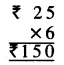Question 2.
₹ 30 × 7
Solution: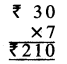Question 3.
₹49 × 8
Solution: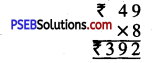Question 4.
₹ 175 × 8
Solution: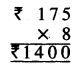Question 5.
₹ 400 × 5
Solution: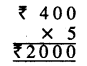Question 6.
₹ 312 × 3
Solution: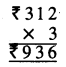Question 7.
₹ 27 × 15
Solution: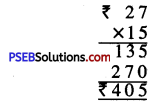Question 8.
₹ 48 × 76
Solution: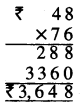Question 9.
₹ 82 × 67
Solution: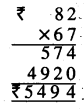Question 2.
The cost of a doll is ₹ 70. Find the cost of 5 such dolls.
Solution:
Cost of.4oll = ₹ 70
Cost of 5 dolls = ₹ 70 × 5
= ₹ 350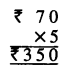Question 3.
Manveet bought a jacket of ₹ 460. Find the cost of 9 such jackets.
Solution:
Cost of jacket = ₹ 460
Cost of 9 jackets = ₹ 460 × 9 = ₹ 4,140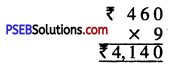Question 4.
Sukhdev bought 35 balloons ₹ 15 per balloon. How much did he pay to the balloon seller ?
Solution:
Cost of 1 balloon = ₹ 15
Cost of 35 balloons = ₹ 15 × 35 = ₹ 525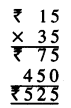Question 5.
The cost of a banana is ₹ 8. What is the cost a dozen bananas ?
Solution:
Cost of 1 banana = ₹ 8
Cost of 1 dozen i.e. 12 bananas = ₹ 8 × 12
= ₹ 96.